Cones and conics - pictures

In the RP2 model of projective geometry,
cones arise from conics in an embedding plane.

 Definitions Given a point O, a line L through O, and αε(0,π/2), the cone with vertex O, axis L and angle α consists of all points on lines through O making angle α with L. The lines are called the generators of the cone. As an example, take the origin O as vertex, the z-axis as axis, and α = π/4. This defines the cone K0 with equation x2+y2=z2. Sections of K0 by planes z = k (k ≠ 0) are circles.Intersections with planes not through the vertex are the usual plane conics. Note. It is far from clear that these are conics as described by the usual focus-directrix definition.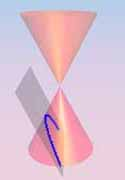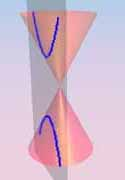ellipse parabola hyperbola Intersections with planes through the vertex give degenerate plane conics. Observe that each is either finite (a single point!), or contains a line (a generator of K0). On the other hand, it is easy to see that no non-degenerate conic contains a line. Theorem A plane conic is non-degenerate if it contains of at least two points, and does not contain 3 collinear points.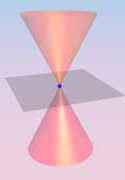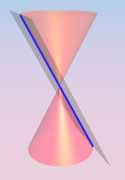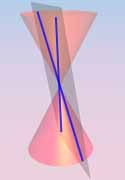point line line pair